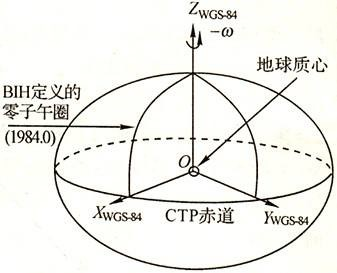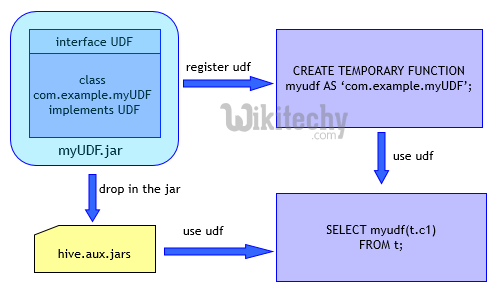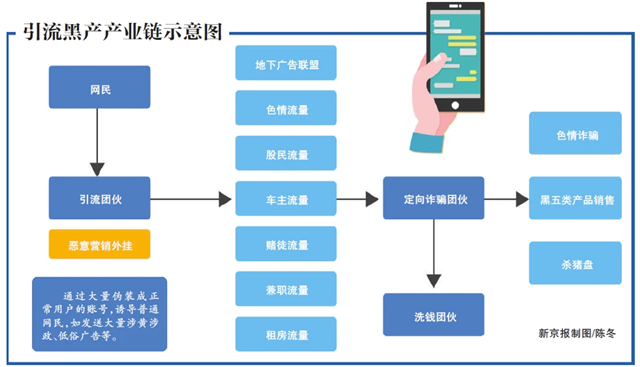# 经纬度与坐标系转换

## WGS-84坐标系

WGS-84坐标系是一种国际上采用的地心坐标系。坐标原点为地球质心，其地心空间直角坐标系的Z轴指向BIH （国际时间服务机构）1984.0定义的协议地球极（CTP)方向，X轴指向BIH 1984.0的零子午面和CTP赤道的交点，Y轴与Z轴、X轴垂直构成右手坐标系，称为1984年世界大地坐标系统。GPS广播星历是以WGS-84坐标系为根据的。

WGS-84采用的椭球是国际大地测量与地球物理联合会第17届大会大地测量常数推荐值，其四个基本参数：

• 长半径：a=6378137±2（m）；
• 地球引力和地球质量的乘积：GM=3986005×108m3s-2±0.6×108m3s-2；
• 正常化二阶带谐系数：C20=-484.16685×10-6±1.3×10-9；
• 地球重力场二阶带球谐系数：J2=108263×10-8
• 扁率f=0.003352810664

## GCJ-02坐标系

GCJ-02是由中国国家测绘局制订的地理信息系统的坐标系统。它是一种对经纬度数据的加密算法，即加入随机的偏差。国内出版的各种地图系统（包括电子形式），必须至少采用GCJ-02对地理位置进行首次加密。

《中华人民共和国地图管理条例》要求地图不得“危害国家统一、主权和领土完整；危害国家安全、损害国家荣誉和利益；国家秘密；影响民族团结、侵害民族风俗习惯”，规定互联网地图服务必须经过审批，要求“从事互联网地图服务的，应当将存放地图数据的服务器设在中华人民共和国境内，建立互联网地图数据安全管理制度和保障措施，并具有经测绘行政主管部门考核合格的互联网地图安全审校人员。”由于地图涉及“国家机密”，中国官方要求地图服务商加装“国家保密插件”，以“保障国家安全”。此插件会将真实的坐标加密成虚假的坐标，且此加偏并非线性加偏，所以各地的偏移情况都会有所不同。

GCJ-02坐标系最关键的地方，概括起来：如果说WGS-84坐标系是地球地理表面某一点真实的经纬度坐标系，那么GCJ-02坐标系是在WGS-84坐标系基础上，把WGS-84真实的经纬度坐标点随机添加一定经纬度偏移量（偏移量计算算法相当复杂，不是简单的随机）而形成的“不真实”的经纬度坐标系。GCJ-02坐标系因此也被称为火星坐标系。

GCJ02加密算法是一种多项式+正弦函数加密算法，通常隔一段时间就换一下参数，所以，网上所谓的解密算法并不是官方的精确算法，隔一段时间说不定就不准确了。有关WGS84和GCJ02，可以查阅参考文档里的《关于GCJ02和WGS84坐标系的一点实验》，出自李民录。

## CGCS2000坐标系

20世纪50年代，为满足测绘工作的迫切需要，中国采用了1954年北京坐标系。1954年之后，随着天文大地网布设任务的完成，通过天文大地网整体平差，于20世纪80年代初中国又建立了1980西安坐标系。1954北京坐标系和1980西安坐标系在中国的经济建设和国防建设中发挥了巨大作用。

2000中国大地坐标系符合 ITRS(国际地球参考系统)的如下定义：

• 原点在包括海洋和大气的整个地球的质量中心；
• 长度单位为米(sI)。这一尺度同地心局部框架的TCG(地心坐标时)时间坐标一致；
• 定向在1984．0时与 BIH(国际时间局)的定向一致；
• 定向随时间的演变由整个地球的水平构造运动无净旋转条件保证。

• 原点 ：地球的质量中心；
• Z轴：指向IERS参考极方向；
• X轴：IERS参考子午面与通过原点且同z 轴正交的赤道面的交线；
• Y轴：完成右手地心地固直角坐标系。

CGCS2000的参考椭球为一等位旋转椭球。等位椭球(或水准椭球)定义为其椭球面是一等位面的椭球。CGCS2000的参考椭球的几何中心与坐标系的原点重合，旋转轴与坐标系的Z轴一致。参考椭球既是几何应用的参考面，又是地球表面上及空间正常重力场的参考面。

• 长半轴a=6378137.0m；
• 扁率f=1/298.257222101；
• 地球的地心引力常数 (包含大气层)GM = 3986004.418×E8m3s-2；

CGCS2000坐标系与WGS84坐标系的差异

• CGCS2000，该系统以ITRF(国际协议地球参考框架)97参考框架为基准，参考框架历元为0。当前，国际地球参考系（ITRS）和国际地球参考框架（ITRF）是世界上最精确、最权威的地心大地坐标系
• 它们都是地心坐标系，坐标原点都在(包含地球周围大气的)地球质心
• 它们的初始参数都来源于GRS(1980)椭球；
• 大地坐标系有4个主要几何参数，两者有3个相同，分别是长半轴，地心引力常数，自转角速度。只有扁率f不同，CGCS2000是f=1/298.257222101，WGS84是1/298.257223563。由此看出两者之间参数定义的区别是很小的，而这一点区别到底有多影响呢，程院长的论文（《2000 国家大地坐标系椭球参数与GRS80和WGS84的比较》）给出的一个数字是：“给定点位在某一框架和某一历元下的空间直角坐标，投影到CGCS2000椭球和WGS84椭球上所得的纬度的最大差异相当于11mm。”
• CGCS2000的定义与WGS84采用的参考椭球非常接近。扁率差异引起椭球面上的纬度和高度变化最大达1mm。当前测量精度范围内，可以忽略这点差异。可以说两者相容至cm级水平，但若一点的坐标精度达不到cm水平，则不认为CGCS2000和WGS84的坐标是相容的。
• 高精度地心坐标必须考虑板块运动影响，由于地球内部地壳运动，坐标参考框架都是动态维持的，其参数需要定期更新。

## BD09坐标系

BD09为百度坐标系，在GCJ02坐标系基础上再次加密。其中BD09LL表示百度经纬度坐标，BD09MC表示百度墨卡托米制坐标。

## 地图服务商的坐标系

• 境内（包括港澳台）：BD09
• 在GCJ-02坐标系基础上再次加密。支持WGS-84、GCJ-02转换成BD09，反向不支持，并且批量转换一次有条数限制
• 境外：WGS-84

• 境内：GCJ-02
• WGS-84 -> GCJ-02（高德有接口提供，反过来没有）
• 境外：暂不支持
• AMap 就是高德地图，是高德地图在纳斯达克上市用的名字，主要面向互联网企业或个人提供免费API服务
• MapABC 是高德集团底下的图盟公司，主要面向大众型企业或政府机关，并提供付费的有偿服务
• Amap和MapABC，数据和服务都是共享的，所以Mapabc用Amap的API是正常的

• 境内：GCJ-02
• 数据来源于高德，两者互通
• 境外：WGS-84

• 全球统一：CGCS2000，国家大地坐标系

• 境内：GCJ02

• 全球统一：WGS-84

• 境内：搜狗坐标系
• 在GCJ-02坐标系基础上再次加密
• 支持WGS-84、GCJ-02、BD09转换成搜狗坐标，反向不支持

• 境内：图吧坐标系
• 在GCJ-02坐标系基础上再次加密

• 境内：GCJ-02

• 境内：GCJ-02

## 不同坐标系之间的转化

### 地球坐标转化成火星坐标

//
// Copyright (C) 1000 - 9999 Somebody Anonymous
// NO WARRANTY OR GUARANTEE
//

using System;

namespace Navi
{
class EvilTransform
{
const double pi = 3.14159265358979324;

//
// Krasovsky 1940
//
// a = 6378245.0, 1/f = 298.3
// b = a * (1 - f)
// ee = (a^2 - b^2) / a^2;
const double a = 6378245.0;
const double ee = 0.00669342162296594323;

//
// World Geodetic System ==> Mars Geodetic System
public static void transform(double wgLat, double wgLon, out double mgLat, out double mgLon)
{
if (outOfChina(wgLat, wgLon))
{
mgLat = wgLat;
mgLon = wgLon;
return;
}
double dLat = transformLat(wgLon - 105.0, wgLat - 35.0);
double dLon = transformLon(wgLon - 105.0, wgLat - 35.0);
double radLat = wgLat / 180.0 * pi;
magic = 1 - ee * magic * magic;
double sqrtMagic = Math.Sqrt(magic);
dLat = (dLat * 180.0) / ((a * (1 - ee)) / (magic * sqrtMagic) * pi);
dLon = (dLon * 180.0) / (a / sqrtMagic * Math.Cos(radLat) * pi);
mgLat = wgLat + dLat;
mgLon = wgLon + dLon;
}

static bool outOfChina(double lat, double lon)
{
if (lon < 72.004 || lon > 137.8347)
return true;
if (lat < 0.8293 || lat > 55.8271)
return true;
return false;
}

static double transformLat(double x, double y)
{
double ret = -100.0 + 2.0 * x + 3.0 * y + 0.2 * y * y + 0.1 * x * y + 0.2 * Math.Sqrt(Math.Abs(x));
ret += (20.0 * Math.Sin(6.0 * x * pi) + 20.0 * Math.Sin(2.0 * x * pi)) * 2.0 / 3.0;
ret += (20.0 * Math.Sin(y * pi) + 40.0 * Math.Sin(y / 3.0 * pi)) * 2.0 / 3.0;
ret += (160.0 * Math.Sin(y / 12.0 * pi) + 320 * Math.Sin(y * pi / 30.0)) * 2.0 / 3.0;
return ret;
}

static double transformLon(double x, double y)
{
double ret = 300.0 + x + 2.0 * y + 0.1 * x * x + 0.1 * x * y + 0.1 * Math.Sqrt(Math.Abs(x));
ret += (20.0 * Math.Sin(6.0 * x * pi) + 20.0 * Math.Sin(2.0 * x * pi)) * 2.0 / 3.0;
ret += (20.0 * Math.Sin(x * pi) + 40.0 * Math.Sin(x / 3.0 * pi)) * 2.0 / 3.0;
ret += (150.0 * Math.Sin(x / 12.0 * pi) + 300.0 * Math.Sin(x / 30.0 * pi)) * 2.0 / 3.0;
return ret;
}
}
}


### 百度坐标与火星坐标的互换

#include <math.h>

const double x_pi = 3.14159265358979324 * 3000.0 / 180.0;

void bd_encrypt(double gg_lat, double gg_lon, double &bd_lat, double &bd_lon)
{
double x = gg_lon, y = gg_lat;
double z = sqrt(x * x + y * y) + 0.00002 * sin(y * x_pi);
double theta = atan2(y, x) + 0.000003 * cos(x * x_pi);
bd_lon = z * cos(theta) + 0.0065;
bd_lat = z * sin(theta) + 0.006;
}

void bd_decrypt(double bd_lat, double bd_lon, double &gg_lat, double &gg_lon)
{
double x = bd_lon - 0.0065, y = bd_lat - 0.006;
double z = sqrt(x * x + y * y) - 0.00002 * sin(y * x_pi);
double theta = atan2(y, x) - 0.000003 * cos(x * x_pi);
gg_lon = z * cos(theta);
gg_lat = z * sin(theta);
}


### 常用地图坐标系转换代码(Python版本)

#! /usr/bin/env python3
# -*- coding:utf-8 -*-

import math

x_pi = 3.14159265358979324 * 3000.0 / 180.0
pi = 3.1415926535897932384626  # π
a = 6378245.0  # 长半轴
ee = 0.00669342162296594323  # 扁率

def gcj02_to_bd09(lng, lat):
"""
火星坐标系(GCJ-02)转百度坐标系(BD-09)
谷歌、高德——>百度
:param lng:火星坐标经度
:param lat:火星坐标纬度
:return:
"""
z = math.sqrt(lng * lng + lat * lat) + 0.00002 * math.sin(lat * x_pi)
theta = math.atan2(lat, lng) + 0.000003 * math.cos(lng * x_pi)
bd_lng = z * math.cos(theta) + 0.0065
bd_lat = z * math.sin(theta) + 0.006
return [bd_lng, bd_lat]

def bd09_to_gcj02(bd_lon, bd_lat):
"""
百度坐标系(BD-09)转火星坐标系(GCJ-02)
百度——>谷歌、高德
:param bd_lat:百度坐标纬度
:param bd_lon:百度坐标经度
:return:转换后的坐标列表形式
"""
x = bd_lon - 0.0065
y = bd_lat - 0.006
z = math.sqrt(x * x + y * y) - 0.00002 * math.sin(y * x_pi)
theta = math.atan2(y, x) - 0.000003 * math.cos(x * x_pi)
gg_lng = z * math.cos(theta)
gg_lat = z * math.sin(theta)
return [gg_lng, gg_lat]

def wgs84_to_gcj02(lng, lat):
"""
WGS84转GCJ02(火星坐标系)
:param lng:WGS84坐标系的经度
:param lat:WGS84坐标系的纬度
:return:
"""
if out_of_china(lng, lat):  # 判断是否在国内
return lng, lat
dlat = _transformlat(lng - 105.0, lat - 35.0)
dlng = _transformlng(lng - 105.0, lat - 35.0)
radlat = lat / 180.0 * pi
magic = 1 - ee * magic * magic
sqrtmagic = math.sqrt(magic)
dlat = (dlat * 180.0) / ((a * (1 - ee)) / (magic * sqrtmagic) * pi)
dlng = (dlng * 180.0) / (a / sqrtmagic * math.cos(radlat) * pi)
mglat = lat + dlat
mglng = lng + dlng
return [mglng, mglat]

def gcj02_to_wgs84(lng, lat):
"""
GCJ02(火星坐标系)转GPS84
:param lng:火星坐标系的经度
:param lat:火星坐标系纬度
:return:
"""
if out_of_china(lng, lat):
return lng, lat
dlat = _transformlat(lng - 105.0, lat - 35.0)
dlng = _transformlng(lng - 105.0, lat - 35.0)
radlat = lat / 180.0 * pi
magic = 1 - ee * magic * magic
sqrtmagic = math.sqrt(magic)
dlat = (dlat * 180.0) / ((a * (1 - ee)) / (magic * sqrtmagic) * pi)
dlng = (dlng * 180.0) / (a / sqrtmagic * math.cos(radlat) * pi)
mglat = lat + dlat
mglng = lng + dlng
return [lng * 2 - mglng, lat * 2 - mglat]

def bd09_to_wgs84(bd_lon, bd_lat):
lon, lat = bd09_to_gcj02(bd_lon, bd_lat)
return gcj02_to_wgs84(lon, lat)

def wgs84_to_bd09(lon, lat):
lon, lat = wgs84_to_gcj02(lon, lat)
return gcj02_to_bd09(lon, lat)

def _transformlat(lng, lat):
ret = -100.0 + 2.0 * lng + 3.0 * lat + 0.2 * lat * lat + \
0.1 * lng * lat + 0.2 * math.sqrt(math.fabs(lng))
ret += (20.0 * math.sin(6.0 * lng * pi) + 20.0 *
math.sin(2.0 * lng * pi)) * 2.0 / 3.0
ret += (20.0 * math.sin(lat * pi) + 40.0 *
math.sin(lat / 3.0 * pi)) * 2.0 / 3.0
ret += (160.0 * math.sin(lat / 12.0 * pi) + 320 *
math.sin(lat * pi / 30.0)) * 2.0 / 3.0
return ret

def _transformlng(lng, lat):
ret = 300.0 + lng + 2.0 * lat + 0.1 * lng * lng + \
0.1 * lng * lat + 0.1 * math.sqrt(math.fabs(lng))
ret += (20.0 * math.sin(6.0 * lng * pi) + 20.0 *
math.sin(2.0 * lng * pi)) * 2.0 / 3.0
ret += (20.0 * math.sin(lng * pi) + 40.0 *
math.sin(lng / 3.0 * pi)) * 2.0 / 3.0
ret += (150.0 * math.sin(lng / 12.0 * pi) + 300.0 *
math.sin(lng / 30.0 * pi)) * 2.0 / 3.0
return ret

def out_of_china(lng, lat):
"""
判断是否在国内，不在国内不做偏移
:param lng:
:param lat:
:return:
"""
return not (lng > 73.66 and lng < 135.05 and lat > 3.86 and lat < 53.55)

if __name__ == '__main__':
lng = 128.543
lat = 37.065
result1 = gcj02_to_bd09(lng, lat)
result2 = bd09_to_gcj02(lng, lat)
result3 = wgs84_to_gcj02(lng, lat)
result4 = gcj02_to_wgs84(lng, lat)
result5 = bd09_to_wgs84(lng, lat)
result6 = wgs84_to_bd09(lng, lat)

print(result1, result2, result3, result4, result5, result6)


• WGS-84 => GCJ-02的误差均值是9米
• WGS-84 => BD-09的误差均值是6米
• GCJ-02 => WGS-84的误差均值是2米
• GCJ-02 => BD-09的误差均值是9米
• BD-09 =>WGS-84的误差均值是2米
• BD-09 => GCJ-02的误差均值是9米

• 原始坐标系弄错。比如以为自己是GPS坐标，但其实已经是GCJ-02坐标。 解决方案：请确保采集到的数据是哪个坐标体系，需要转换到哪个坐标系，再进行坐标转换。
• 原始坐标准确度不够。解决方案：如果您是GPS坐标，请确保采集GPS数据时，搜到至少4颗以上的卫星。并且GPS数据准不准，还取决于周围建筑物的高度，越高越不准，因为有遮挡。 如果本来就是GCJ-02坐标，在不同地图放大级别的时候，看到的地方可能不一样。比如你在地图级别4（国家）取到的坐标，放大到地图12级（街道）时，坐标就偏了。请确保在地图最大放大级别时，拾取坐标。
• 度分秒的概念混淆。比如，在googleearth上采集到的是39°31’20.51，那么应该这样换算，31分就是31/60度，51秒就是20.51/3600度，结果就是39+ 31/60 + 20.51/3600 度。
• 经纬度顺序写反了。有些公司（比如高德，百度，腾讯）是先经度，再纬度，即Point(lng lat)。但谷歌坐标的顺序恰好相反，是(latlng)。##### Hive UDF的开发简介